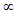## NOTE:

If error occur=> Change website url from chemistrynotesinfo.blogspot.in to

## Structure of Atom

### Atomic Number

Number of protons present in nucleus of atom of an element is known as atomic number.
It is denoted by (z)
example : Carbon have 6 protons so its atomic number is 6 (z = 6)
For neutral atom,
atomic number (z) = number of protons (p) = number of electron (e)

Number of protons in cation > Number of electrons in cation
Number of protons in anion < Number of electrons in anion
Number of protons in neutral atom = Number of electron in neutral atom

### Mass Number

Mass number is also known as atomic mass. Atomic number or mass number is equal to number of protons and neutrons in atom.
Atomic mass = number of protons + number of neutrons
A = p+n
example : Helium have two protons and two neutrons so its atomic mass is 2+2=4

### Isotopes

Atoms of same elements which have same atomic number but different mass number is called isotopes.
example : Protium, Deutrium, Tritium are isotopes of hydrogen.

#### Types of Isotopes

1. Non-radioactive isotope example C(12), C(13)
2. Radioactive isotopes example U(235), U(233), U(238), U(239)

### Isobars

Atoms of different elements which have similar atomic mass and different atomic number are called isobars.
example : Ca(40), Ar(40), Kr(40)
50Cr24, 50Ti22
Isobars do not similar in physical and chemical properties

#### Distribution of electrons in various orbits : ( Bohr Bury  Scheme )

In 1921, Bohr and Bury gives some laws for distribution of electrons in various orbits, which are called as Bohr-Bury Scheme.
Rules of Bohr Bury scheme are as follows-
1. Maximum number of electron in any shell should be 2n2 , where n is serial number of shell.
2. Maximum number of electron that exist in outermost shell or orbit of any atom is eight while maximum number is eighteen for penultimate shell.
3. According to this law, it is not necessary that another shell is formed after completion of 1st shell. A new shell is formed as number of electrons reaches eight in any orbit or shell.

### Valence Electrons and Valency

Outermost incomplete shell of any atom is known as Valence Shell and electron present in incomplete outermost shell are known as Valence Electrons , which participate in formation of bond.
• If atoms have 1 to 4 valence electrons then VALENCY = Number of valence electron
• If atoms have 5 to 8 valence electrons then VALENCY = 8 - Number of valence electron
• But these above rule is not applicable to atoms which show variable valency

Radioactivity is discovered by French scientist Becquerel in 1896. Substances which emits radiation is known as radioactive substances and this property of substances to emit radiation is called as radioactivity.

Rutherford conduct some experiments with radioactive substances and his observations are as follows-
1. Rays which turned toward negative electrode is known as alpharays, mass of alpha particles is 4 a.m.u.  and charge is 2 units of positive charge.
2. Rays which turned toward positive electrode is known as betarays, mass and charge of beta particle is equal to mass and charge of electron.
3. The rays which do not turned towards any electrode is known as gammarays, gamma rays are electromagnetic rays and not made from matter particle.

#### Penetrating Power

Gamma > Beta > Alpha
Gamma rays have maximum penetrating power while Alpha has lowest.

### Tracer technique of radioactive isotopes

As per requirement, small amount of radioactive isotopes is added to its element then many physical and chemical complex reaction are studied.

### Various fields of tracer techniques

1. In the field of chemical science.
2. In the field of medical science.
3. In the field of agriculture.
4. In the industrial fields.
5. And in other scientific fields.

#### Carbon Dating

In this technique carbon isotope is used to determine the age of rocks, minerals, fossils, dead animals and dead plants.